﻿ 基于POI数据的扬州市消费服务设施空间格局分析

# 基于POI数据的扬州市消费服务设施空间格局分析Spatial Pattern Analysis of Consumer Service Facilities in Yangzhou Based on POI Data

Abstract: The consumption service facilities (CSFs) are the important engine of urban economy development. This study selected the point of interest (POI) data, and adopted the methods of coefficient of variation, average nearest neighbor index, kernel density analysis and correlation analysis to analyze the spatial characteristics of the CSFs of Yangzhou based on the spatial data analysis tool. The spatial distribution and the influence factors of the CSFs were concluded. The results are as follows: 1) The spatial distribution of consumer service facilities in Yangzhou is characterized by aggregation. 2) The distribution differences of various consumer service facilities in the selected research area are relatively obvious. 3) The population density, the road cross node density and the road network density have good correlation with the distribution density of consumption service facility.

1. 引言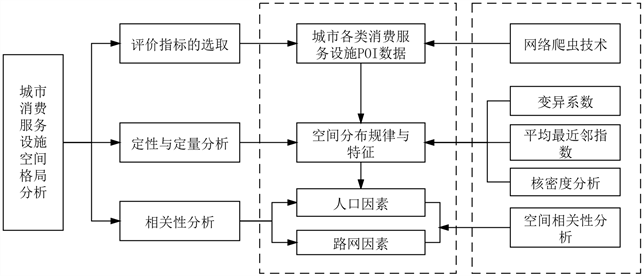Figure 1. Research framework

2. 数据与方法

2.1. 研究区域和数据来源

2.2. 数据来源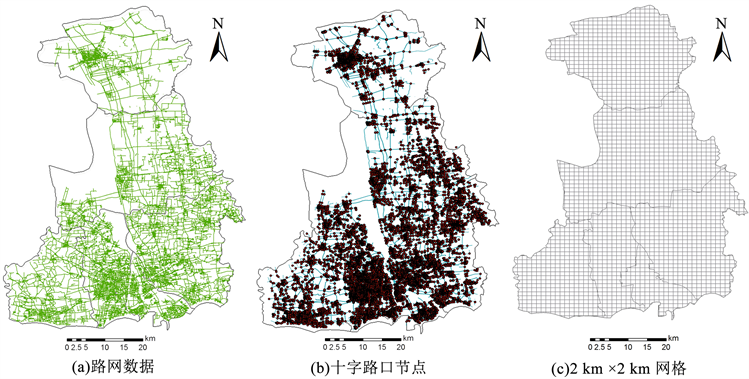Figure 2. Yangzhou road network raw dataTable 1. POI consumption service facilities of the districts and counties in YangzhouTable 2. Total population, density and area of the districts and counties in Yangzhou (2019)

2.3. 研究方法

1) 变异系数分析

${C}_{v}=\frac{\sigma }{\mu }=\frac{\sqrt{{\sum }_{i=1}^{n}{\left({X}_{i}-\stackrel{¯}{X}\right)}^{2}}}{n\stackrel{¯}{X}}$ (1)

2) 平均最近邻分析

$ANN=\frac{{\stackrel{¯}{D}}_{O}}{{\stackrel{¯}{D}}_{E}}=\frac{\frac{{\sum }_{i=1}^{n}{d}_{i}}{n}}{\frac{0.5}{\sqrt{n/A}}}$ (2)

Z得分是用来检验空间自相关分析的统计显著性，在该分析中，Z得分为负代表集聚，为正代表发散。平均最近邻Z得分的计算方式为：

$Z=\frac{{\stackrel{¯}{D}}_{O}-{\stackrel{¯}{D}}_{E}}{SE}=\frac{{\stackrel{¯}{D}}_{O}-{\stackrel{¯}{D}}_{E}}{\frac{0.26136}{\sqrt{{n}^{2}/A}}}$ (3)

3) 核密度分析

${\stackrel{^}{f}}_{h}\left(x\right)=\frac{1}{n}\underset{i=1}{\overset{n}{\sum }}{k}_{h}\left(x-{x}_{i}\right)=\frac{1}{nh}\underset{i=1}{\overset{n}{\sum }}k\left(\frac{x-{x}_{i}}{h}\right)$ (4)

4) 相关性分析

${r}_{xy}=\frac{{\sum }_{i=1}^{n}\left({x}_{i}-\stackrel{¯}{x}\right)\left({y}_{i}-\stackrel{¯}{y}\right)}{\sqrt{{\sum }_{i=1}^{n}{\left({x}_{i}-\stackrel{¯}{x}\right)}^{2}}\sqrt{{\sum }_{i=1}^{n}{\left({y}_{i}-\stackrel{¯}{y}\right)}^{2}}}$ (5)

3. 消费服务设施的空间格局分析

3.1. 消费服务设施的空间集聚Figure 3. Statistics of coefficient of variation of various POI consumer service facilities in Yangzhou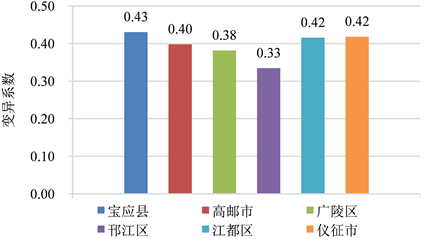Figure 4. Statistics of variation coefficient of consumer service facilities at POI points in each district and county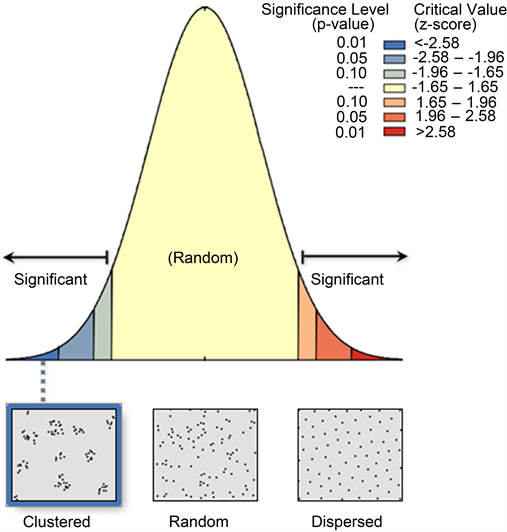Figure 5. Summary of average nearest neighbor of urban consumer service facilities in YangzhouTable 3. Average nearest neighbor calculations for various POI consumer service facilities

3.2. 消费服务设施的空间特征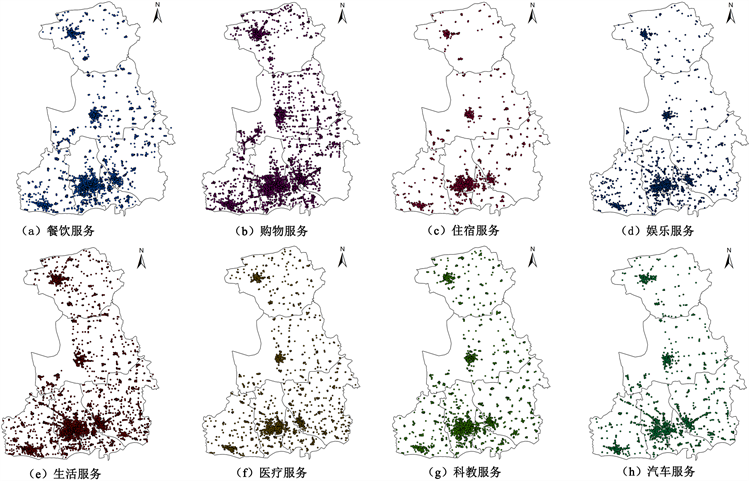Figure 6. Distribution of POI points of various consumer services facilities in Yangzhou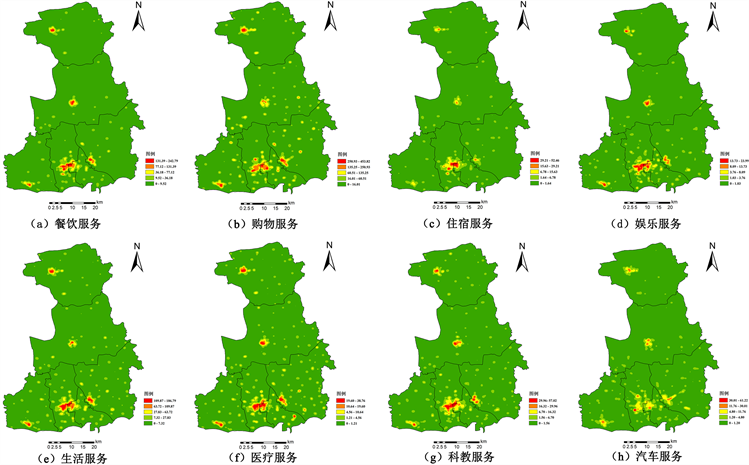Figure 7. Nuclear density distribution of various consumer service facilities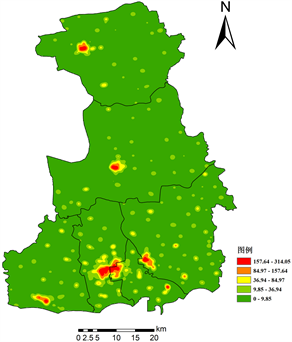Figure 8. Nuclear density distribution of consumer services facilities in Yangzhou

4. 消费服务设施空间分布的影响因素分析

4.1. 人口密度与消费服务设施密度的相关性分析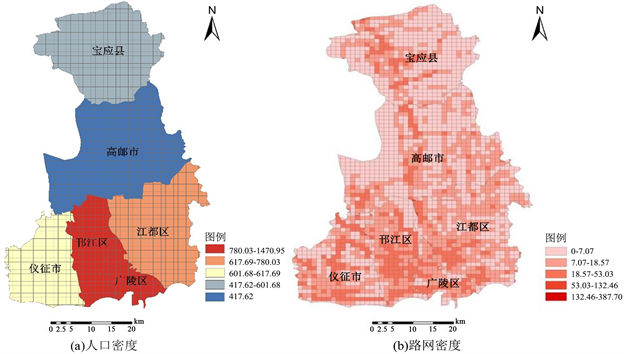Figure 9. Population and road network density analysisTable 4. Correlation between population density and density of consumer service facilities

**.在0.01级别(双尾)，相关性显著。

4.2. 路网密度与消费服务设施密度的相关性分析Table 5. Correlation between road cross node density and consumer service facility density

**.在0.01级别(双尾)，相关性显著。Table 6. Correlation between road network density and density of consumer service facilities

**.在0.01级别(双尾)，相关性显著。

5. 研究结论

1) 总体而言，扬州市城市消费服务设施在整体空间分布上呈现一定的集聚状态，并呈现南高北低的峰值集聚，其中邗江区密度分布最高，城市消费服务设施较为完善，对居民产生较强的消费吸引。

2) 在各区的分布差异上，不同区域内消费服务设施数量分布差异比较明显，宝应县内各类消费服务设施数量相差较大，邗江区内各类消费服务设施数量相差最小；在空间分布特征上，各类型消费服务设施都表现出一定的集聚倾向，其中购物、餐饮、生活三类消费服务设施分布密度最高，餐饮服务设施的分布特征是最集中的。

3) 从影响因素方面来看，人口密度、道路十字节点密度、道路网密度与消费服务设施分布密度之间呈正相关性，相关性显著，这说明交通系统的发达性为居民的出行提供便利。

 张晶宇, 范熙晅. 基于POI数据的城市服务设施分布与空间格局研究——以大连市中心城区为例[J]. 建筑与文化, 2020(12): 74-75.

 李卫东, 张铭龙, 段金龙. 基于POI数据的南京市空间格局定量研究[J]. 世界地理研究, 2020, 29(2): 317-326.

 路邯淞, 倪修宝, 刘科彬. 消费行为视角下的城市农贸市场服务空间分析——以宿迁市中心城区为例[J]. 江苏师范大学学报(自然科学版), 2016, 34(1): 12-14+23.

 綦慧心. 基于服务与交通网络视角的城市消费空间活力分析[J]. 商业经济研究, 2019(8): 48-51.

 路旭, 李古月. 基于空间网络格局的城市商业服务设施分布特征研究[J]. 华中建筑, 2020, 38(8): 95-99.

Top